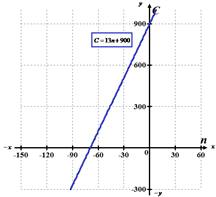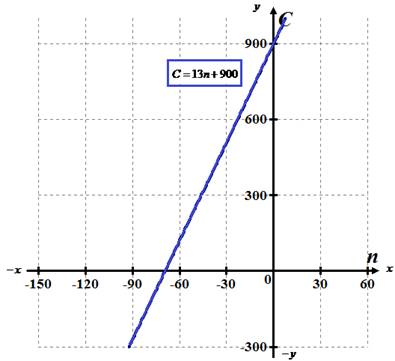# Assuming that the relationship between cost and number of chairs produced is linear, find an equation that expresses this relationship. Then graph the equation.### Precalculus: Mathematics for Calcu...

6th Edition
Stewart + 5 others
Publisher: Cengage Learning
ISBN: 9780840068071### Precalculus: Mathematics for Calcu...

6th Edition
Stewart + 5 others
Publisher: Cengage Learning
ISBN: 9780840068071

#### Solutions

Chapter 1.10, Problem 76E

a.

To determine

## Assuming that the relationship between cost and number of chairs produced is linear, find an equation that expresses this relationship. Then graph the equation.

Expert Solution

C=13n+900### Explanation of Solution

Calculation:

Assume that the relationship between cost and number of chairs produced is linear, and then the equation that represents this relationship is given as,

C=mn+b

To manufacture 100 chairs, it costs $2200 . So, substitute n=100 and C=2200 in the equation C=mn+b , we get, 2200=m(100)+b2200=100m+b............(1) To manufacture 300 chairs, it costs$4800 . So, substitute n=300 and C=4800 in the equation C=mn+b , we get,

4800=m(300)+b4800=300m+b............(2)

Subtract equation (2) from equation (1) ,

4800=300m+b2200=100mb2600=200m13=mm=13

So, the value of m is m=13 .

Now, we substitute the value m=13 in equation (1) , we get:

2200=100(13)+b2200=1300+bb=900

Put these values of m=13 and b=900 in C=mn+b to get,

C=13n+900

So, the linear equation that expresses this relationship between cost and number of chairs produced is C=13n+900 .

The graph of the equation C=13n+900 is given below,Hence, the linear equation that expresses this relationship between cost and number of chairs produced is C=13n+900 and the graph is shown above.

b.

To determine

### What is the slope of the line in part (a) and what does it represent?

Expert Solution

13

It represents the cost per number of chairs.

### Explanation of Solution

Calculation:

Since, the equation of the line that has slope m and yinterceptb is y=mx+b .

Hence, the slope of the line C=13n+900 is 13 and it represents the cost per number of chairs.

c.

To determine

### What is y−intercept of this line, what does it represent?

Expert Solution

900

It represents the monthly fixed cost.

### Explanation of Solution

Calculation:

Since, the equation of the line that has slope m and yinterceptb is y=mx+b .

Hence, the yintercept of the line C=13n+900 is 900 and it represents the monthly fixed cost.

### Have a homework question?

Subscribe to bartleby learn! Ask subject matter experts 30 homework questions each month. Plus, you’ll have access to millions of step-by-step textbook answers!